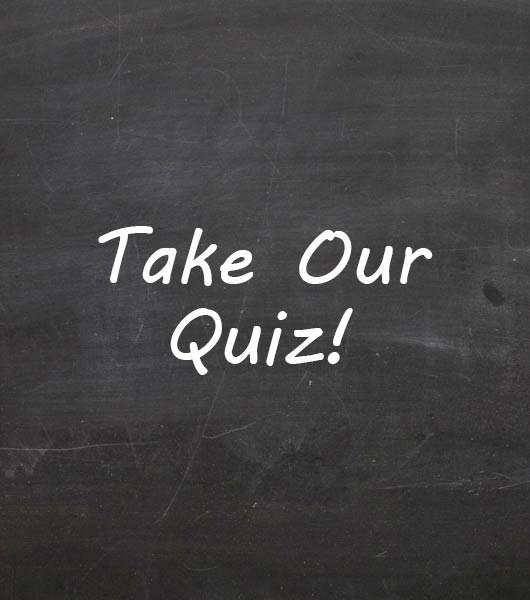# Stem Ch 2 Online Test

22 Questions | Attempts: 224SettingsCovers basic chemistry and water

• 1.
Atoms are composed of
• A.

Protons with a positive charge

• B.

Neutrons with no charge

• C.

Electrons with a negative charge

• D.

All of the above

• 2.
Electrons of an atom
• A.

Are found in the nucleus along with the protons

• B.

Orbit the nucleus in various energy levels

• C.

Have a positive charge

• D.

Are attracted to the positive charge of neutrons

• 3.
The bond formed when two atoms share a pair of electrons is called a
• A.

Hydrogen bond

• B.

Nonpolar bond

• C.

Covalent bond

• D.

Ionic bond

• 4.
An atom that has gained or lost electrons is called a (an)
• A.

Molecule

• B.

Quark

• C.

Ion

• D.

Element

• 5.
Atoms become more stable when they form compounds because
• A.

They always lost electrons when they form compounds

• B.

Their outer orbitals become filled when they form compounds

• C.

They always gain electrons when they form compounds

• D.

Their nucleus loses extra protons when they form compounds

• 6.
A water molecule is polar because its hydrogen and oxygen atoms
• A.

Both lose electrons

• B.

Become ions

• C.

Both gain electrons

• D.

Do not share the electrons equally

• 7.
Polar molecules, such as water, have
• A.

No negative and positive poles

• B.

Both negative and positive poles

• C.

Only a negative pole

• D.

Only a positive pole

• 8.
Which of the following characteristics of water is NOT a result of hydrogen bonding?
• A.

• B.

Capillarity

• C.

Cohesion

• D.

All of the above are a result of hydrogen bonding

• 9.
Is HCl an ionic or covalent compound?
• A.

Ionic

• B.

Covalent

• C.

Neither

• D.

Both

• 10.
When drawing a Bohr diagram, how many electrons can fit on the inner orbital closest to the nucleus?
• A.

1

• B.

2

• C.

4

• D.

8

• 11.
On a Bohr diagram, the electrons on the outermost shell are called...
• A.

Ions

• B.

Valence electrons

• C.

Isotopes

• D.

Electronegative

• 12.
If you were to draw the Bohr diagram for Sulfur, how many protons would be on the diagram?
• A.

6

• B.

10

• C.

16

• D.

32

• 13.
On the Bohr diagram of Sulfur, how many orbitals (shells) would the drawing contain?
• A.

1

• B.

2

• C.

3

• D.

4

• 14.
On a Lewis Dot diagram of Sulfur, how many dots would you draw around the S?
• A.

3

• B.

6

• C.

16

• D.

32

• 15.
How many neutrons does Chlorine have?
• A.

10

• B.

17

• C.

18

• D.

34

• 16.
If you were to bond Magnesium with Fluorine to make magnesium fluoride, how would you write the final compound out with charges?(Imagine the numbers on the compound choices are subscripts)
• A.

MgF2 with Mg having a charge of +2 and Fluorine having a charge each of -1.

• B.

Mg2F with Mg having a charge each of -1 and Fluorine having a charge of +2

• C.

MgF no charges on either because it is a covalent bond

• D.

F2Mg with Fluorine having a charge each of +1 and Magnesium having a charge of -2.

• 17.
If you were to bond hydrogen with bromine, would the bond between them be single or a double bond?
• A.

Single

• B.

Double

• C.

Both

• D.

Neither

• 18.
Which property of water explains the fact that a penny can hold a lot of water drops?
• 19.
How many electrons does fluorine need to gain or lose in order to fill its outer shell?
• 20.
Give a specific example of a cation.
• 21.
Imagine sucrose has the formula C12H22O11.  (The numbers are subscripts here).  How many atoms does oxygen contain?
• 22.
What is the atomic mass number of carbon?

## Related TopicsBack to top